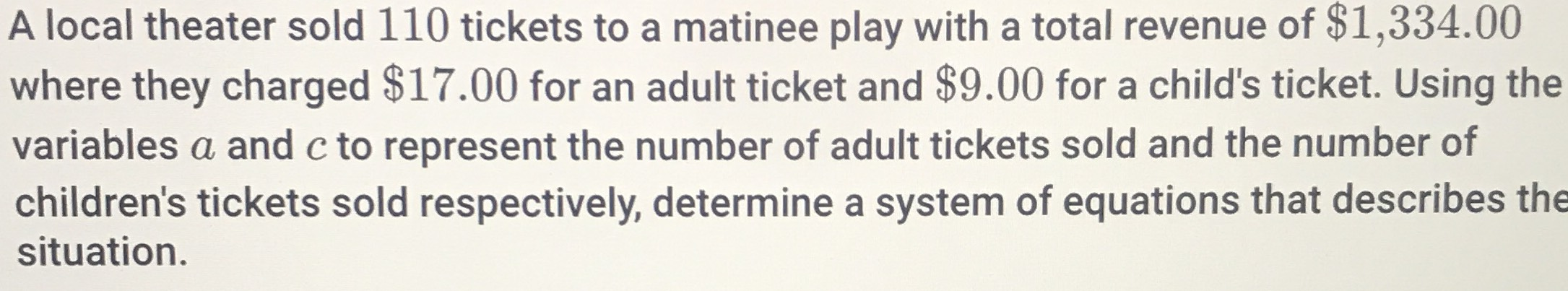### Still have math questions?

Algebra
QuestionA local theater sold $$110$$ tickets to a matinee play with a total revenue of $$\ 1,334.00$$

where they charged $$\ 17.00$$ for an adult ticket and $$\ 9.00$$ for a child's ticket. Using the variables $$a$$ and $$c$$ to represent the number of adult tickets sold and the number of children's tickets sold respectively, determine a system of equations that describes the situation.